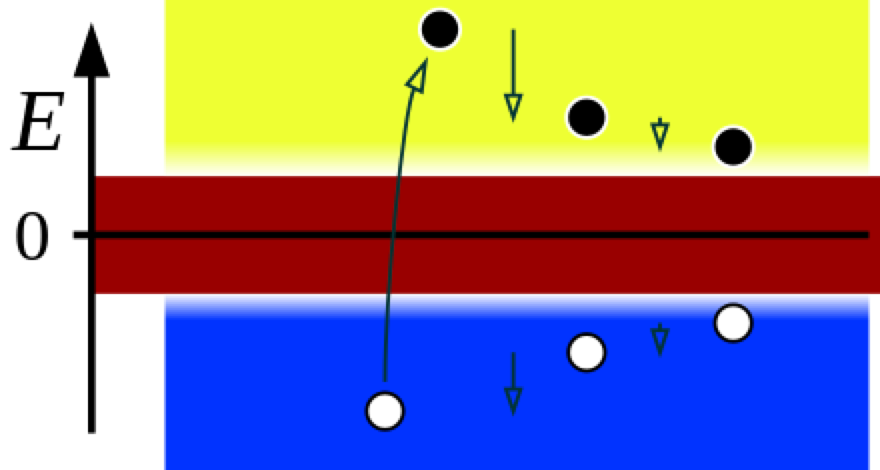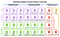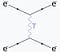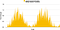Figure 1, Dirac sea for massive particles and anti-particles

The Karpinsky Interpretation of Quantum Mechanics (Part 2)

Introduction

As described in Part 1, the Karpinsky Interpretation of Quantum mechanics is a purely wave theory. All interactions are the interaction of one wave with another wave. This follows from the mathematical formalism of quantum mechanics. The Schrodinger equation is a wave equation, and there is no reason to claim that what is being described is a particle. We will occasionally still use the word “particle” for waves that have enough energy to meet the threshold required for existence, but that is only because the language is already there.

The Mass Shell Condition

The ‘mass shell” condition is that “Particles” exist only when they have enough energy to exist. That is when the energy is more than or equal to the amount needed for the particle to exist. E= mc² for a massive particle. The standard model of quantum mechanics describes all the known particles and describes them in terms of the energy that is required for them to exist. If the energy of the Higgs field is less than these energies, the particles cannot exist. This is shown in Figure 1 where the shaded area around zero energy is too low to manifest a phase change of the wave to make what we call a particle. In the yellow region, matter can exist, and in the blue region, anti-matter can exist. Figure 2 shows another view of the Dirac sea.Figure 2, Dirac Sea

Figure 3 shows all the particles and their energies. We don’t know why these are the particles or the energies, but from quantum theory and experiment, these are the facts. But one other fact is that all of these are still just waves. I like to call them standing waves in analogy to the standing waves on a vibrating string. For vibrating strings, the length of the string determines what wavelengths are allowed. That is how a guitar works. In a similar way, the laws of the universe determine the effective length of the equivalent of strings, creating the particles in the universe. It is a resonance which we will one day understand. Or maybe we already know why, but some physicist forgot to tell us.Figure 3, All the particles and their energies

There are many interactions of waves that do not meet the mass shell condition. In the Feynman diagrams, these interactions are described as the actions of virtual photons that act on the “particles”. This is a very tortured way of describing these interactions. It is much simpler to simply describe these interactions as the interaction of one wave with another wave.Figure 4, Electron-Electron Scattering

For instance, if two electrons are on paths that are close enough to interact, a Feynman diagram will show that they exchange “virtual photons” to affect each other, as shown in figure 4. This way of describing it is somewhat intuitive but totally unnecessary. The descriptions of fields by Maxwell, Gauss, and Faraday are perfectly adequate descriptions of the interactions. These virtual photons are describing forces due to wave actions where the energy is below the mass shell condition. In this low energy region, the electro-magnetic fields push the particles around.

Photons

Only massive particles are standing waves in free space. Photons travel at the speed of light and they are quantized in only two conditions. One is if they are in an optical cavity where the allowed wavelengths of the photons in the cavity are determined by the distance between the cavity mirrors. When photons interact with massive waves (particles), they can then also be quantized. But the quantization is generated by the quantization of the particles and this is not inherent to the Electro-Magnetic(EM) wave.
Even the blackbody radiation quantization of the photons emitted is a property of the massive particles and not the EM waves. The quantization property of the photo-electric effect is due to the quantization of the electron in an atom, and not a property of the photon. There is a quantization of the photon, but it is not in frequency or energy. It is in the amplitude or field strength of the electric and magnetic fields. All photons must have the same amplitudes. This can easily be seen from the doppler effect. When a photon is emitted by a particle that is moving away from us, the wavelength we see is lengthened from what it was at the emitting particle. But it is the same photon, so it must have the same amplitude that it had when it was emitted. This is inherent in the formula for the energy of a photon. Energy = Planck’s constant times the frequency. There is no amplitude in that formula. So all photon EM amplitudes must be the same. This is true even of the photons emitted in the early universe that we now see as the cosmic microwave background. Even those photons have the same amplitude they had when they were emitted. They have just a much lower frequency.

Quantum Uncertainty

Born’s (1926b) probability law is the basis of the statistical interpretation in the Copenhagen interpretation. Here, P is the probability of the existence of the particle.

The second wave function with the * is the complex conjugate of the wave function. Born’s law has no basis and has no mechanism for the uncertainty. It is just conjecture that matches measurements. However, the Karpinsky interpretation provides the physical basis for why this law describes the probability. It is the quantum foam or fluctuations as shown in Figure 5. It does not change anything about the calculations of quantum mechanics, but it provides a physical basis for interpreting these calculations as probabilities. It also provides an avenue for further investigations into these probabilities. We don’t know the amplitude of the fluctuations or what statistics they follow. I think that there are experiments that can be done to explore these fluctuations which I will now call noise. We don’t know the amplitude of the noise, or even if it is constant in all of the universe. We don’t know the spectral distribution of the noise, but I am going to assume white noise unless it is proven otherwise. If we can measure or calculate these quantities, it will great improve our understanding of the vacuum, or as shown in Figure 1, the Dirac sea.Figure 5, Simulated Sum of Electron Wave and Noisy Higgs Wave Background

Another Interpretation of QM

So far, we have not discussed how to reconcile a photon’s Frame Of Reference(FOR) with our stationary FOR. There is another interpretation that addresses these things in a manner consistent with the Karpinsky interpretation. That is “The Transactional Interpretation of Quantum Mechanics” by John G Cramer (1986) published in Reviews of Modern Physics. Many thanks to Janos Madar, in Medium, for noticing the similarity of The transactional interpretation to the Karpinsky interpretation. The transactional interpretation of quantum mechanics (TIQM) by John G. Cramer is an interpretation of quantum mechanics inspired by the Wheeler–Feynman absorber theory.

The basic element of this interpretation is the transaction, describing a quantum event for photons as an exchange of advanced and retarded waves, as implied by the work of Wheeler and Feynman, Dirac, and others. The transactional interpretation is explicitly nonlocal and thereby consistent with the tests of the Bell inequality, yet is relativistically invariant and fully causal. The transactional interpretation permits quantum-mechanical wave functions to be interpreted as real waves physically present in space rather than as “mathematical representations of knowledge” as in the Copenhagen interpretation. The transactional interpretation is shown to provide insight into the complex character of the quantum-mechanical state vector and the mechanism associated with its “collapse. “ It also leads in a natural way to justification of the Heisenberg uncertainty principle and the Born probability law, which are basic elements of the Copenhagen interpretation.

The transactional interpretation describes a transaction in the mathematical formalism of quantum mechanics. With the advanced and retarded waves, Cramer describes the emission and absorption of a photon in our FOR in a way that is compatible with the instantaneous existence of the photon in the photon’s FOR. What this means is that, in a photon’s FOR, no time passes between the emission and absorption of the photon. But, according to the transactional interpretation, the advance and retarded waves show how that appears in our FOR. The only difference I can find between the two interpretations is that the Karpinsky interpretation posits that the source of the uncertainty in a quantum mechanical measurements is the random fluctuation of the Higgs field.

I am a retired industrial physicist interested in the fundamental physics of the universe.

## More from John Karpinsky

I am a retired industrial physicist interested in the fundamental physics of the universe.